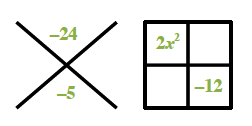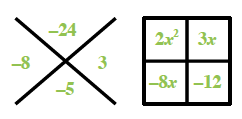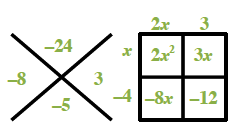### Home > A2C > Chapter 2 > Lesson 2.1.2 > Problem2-28

2-28.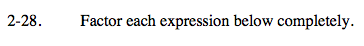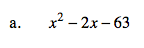Try to think of two factors of 63 that have a difference of 2.

Notice that the constant, −63, is negative.

(x − 9)(x + 7)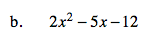Try using a diamond problem and a generic rectangle.

To get 2x2 you need factors (2x )(x ).

(2x + 3)(x − 4)